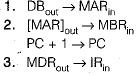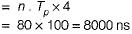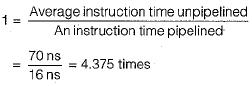Courses

# Pipelining (Basic Level)- 1

## 10 Questions MCQ Test Question Bank for GATE Computer Science Engineering | Pipelining (Basic Level)- 1

Description
This mock test of Pipelining (Basic Level)- 1 for Computer Science Engineering (CSE) helps you for every Computer Science Engineering (CSE) entrance exam. This contains 10 Multiple Choice Questions for Computer Science Engineering (CSE) Pipelining (Basic Level)- 1 (mcq) to study with solutions a complete question bank. The solved questions answers in this Pipelining (Basic Level)- 1 quiz give you a good mix of easy questions and tough questions. Computer Science Engineering (CSE) students definitely take this Pipelining (Basic Level)- 1 exercise for a better result in the exam. You can find other Pipelining (Basic Level)- 1 extra questions, long questions & short questions for Computer Science Engineering (CSE) on EduRev as well by searching above.
QUESTION: 1

### Pipelining improves CPU performance due to

Solution:

In pipelining, a number of functional units are employed in sequence to perform a single computation.

QUESTION: 2

### An instruction cycle refers to

Solution:

An instruction cycle is the basic operational process of a computer. It is the process by which a computer retrieves a program instruction from its memory, determines what actions the instruction dictates and carries out those actions. Also called as fetch, decode-execute cycle.

QUESTION: 3

### Given a 5 stage pipeline with stages taking 1,2, 3, 1, 1 units of time, the clock period of the pipeline is

Solution:

Clock period of pipeline = Maximum delay of stages
= Max ( 1, 2, 3, 1, 1) = 3

QUESTION: 4

Which of following registers processor used for fetch and execute operations?
1. Program counter
2. Instruction register

Solution:

μ-instruction for fetch cycle:So, PC, IR and address register are used.

QUESTION: 5

A ________ is required to translate such microprogram into executable programs that can be stored in the control memory in microprogramming.

Solution:

It is the definition of microassembler.

QUESTION: 6

Which of the following statements is false about CISC architectures?

Solution:

In RISC instruction set all arithmetic/logic instructions must be register-based but not in CISC.

QUESTION: 7

The register which holds the address of the location to or from which data are to be transferred is known as

Solution:

MAR is a register that holds the address of location to or from which data are to be transferred.

QUESTION: 8

Consider a case where 4-segment pipeline with a clock cycle time 20 ns in each sub operation to execute 100 tasks. Assume that a non pipeline unit that can perform the same operation. Pipelined system will take how much time to complete the task?

Solution:

For pipeline with K segment and n instruction
Time (pipeline ) = ( K + n - i ) tP
= ( 4 + 100 - 1 ) x 20 nsec
= (4 + 99) x 20 nsec
= (103) x 20 nsec = 2060 nsec

QUESTION: 9

Find out the speed-up ratio between pipelined and non-pipelined system?

Solution:

The needed for non pipelined processorSpeedup = 8000/2060 = 3.88

QUESTION: 10

Assume that the time required for the eight functional units, which operate in each of the eight cycles, are as follows 5 ns, 8 ns, 6 ns, 10 ns, 15 ns, 12 ns, 6 ns, 8 ns. Assume that pipe lining adds 1 ns of overhead. Find the speedup versus the single cycle data path.

Solution:

Since the unpipelined machine executes all instructions in a single clock cycle, its average time per instruction is simply clock time. The dock is equal to the sum of the times for each step in the execution.
∴ Average instructions execution time
= 5 + 8 + 6 + 1 0 + 15 + 12 + 6 + 8
= 70
The dock cycle time on the pipelined machine must be the largest time for any stage in the pipeline (15 ns) + the overhead of 1 ns for a total of 16 ns.
∴ Speed from pipelining